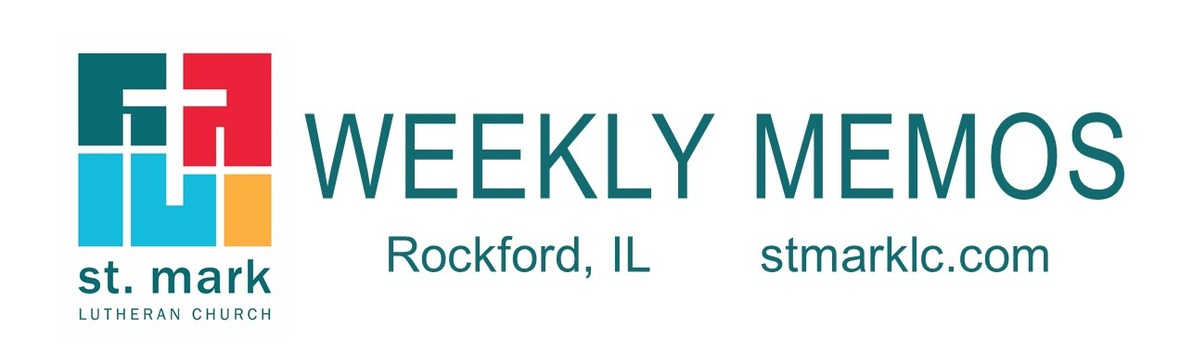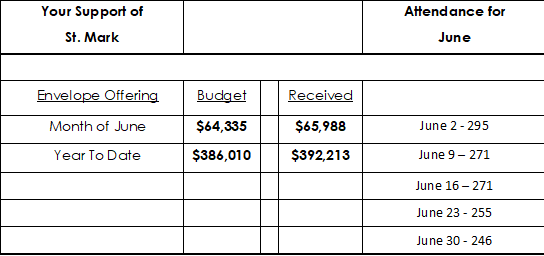Like   Tweettable div table+table div table{width:100%;padding:0}table div table+table div table img{width:96.23%;padding:0;float:none}table div table+table div table td{width:100%;padding:0 1.88% 18px}/* styles */## Pastoral ReflectionWords Can Always Heal You

Words are all around us. We read what is written by friends and journalists online. We hear important information spoken everywhere from the doctor’s office to the dinner table. Most of the words around us have little effect on your life, but every now and then someone says something that can grab your focus and make you see the world in a different way. Read more.

 table div table+table+table+table+table div table{width:100%;padding:0}table div table+table+table+table+table div table img{width:96.23%;padding:0;float:none}table div table+table+table+table+table div table td{width:100%;padding:0 1.88% 18px}/* styles */## Sunday, July 14

8:00 A.M. Worship
9:30 A.M. Worship
9:30 A.M. Baptism of Cameron Ryan
11:00 A.M. Worship
11:00 A.M. Baptism of Leonardo Heffner

## Monday, July 15

6:30 P.M. Emotions Anonymous
6:30 P.M. Blue Lights
6:30 P.M. Theology Pub at Carlyle Brewing Co.

## Tuesday, July 16

10:00 A.M. Staff Meeting
11:30 A.M. Duplicate Bridge

## Wednesday, July 17

9:00 A.M. Lemonade Stand for Childhood Cancer at Edgebrook's Farmers Market
6:30 P.M. Youth Summer Fun Night

## Thursday, July 18

9:00 A.M. Cherry Valley Bridge Group
2:00 P.M. Barnabas Meeting
6:30 P.M. Emotions Anonymous
7:00 P.M. Praise Band Rehearses

## Friday, July 19

7:30 A.M. St. Mark Men's Breakfast at Denny's

## Sunday, July 20

8:00 A.M. Worship
9:30 A.M. Worship
11:00 A.M. Worship

 table div table+table+table+table+table+table+table+table+table div table{width:100%;padding:0}table div table+table+table+table+table+table+table+table+table div table img{width:96.23%;padding:0;float:none}table div table+table+table+table+table+table+table+table+table div table td{width:100%;padding:0 1.88% 18px}/* styles */## News and Events

 table div table+table+table+table+table+table+table+table+table+table+table div table{width:100%;padding:0}table div table+table+table+table+table+table+table+table+table+table+table div table img{width:96.23%;padding:0;float:none}table div table+table+table+table+table+table+table+table+table+table+table div table td{width:100%;padding:0 1.88% 18px}/* styles */We are excited to announce a new Church Directory.

If you have not submitted a photo for the new directory simply follow the link below to easily upload your own photo to insert into the church directory. On your phone? Snap a quick photo of you or your family and upload. Can't wait to see you!
Instant Church Directory

Instant Church Directory is a simple way to view your Church Directory. Never deal with an outdated directory again, any changes made by church staff are immediately synced to the app.

Mobile App Login Instructions for Android & Kindle Devices

We also have a form for you to complete to make sure we have the most current information for the directory. There are paper forms at church or click on the link to Church Directory Form

 table div table+table+table+table+table+table+table+table+table+table+table+table+table div table{width:100%;padding:0}table div table+table+table+table+table+table+table+table+table+table+table+table+table div table img{width:96.23%;padding:0;float:none}table div table+table+table+table+table+table+table+table+table+table+table+table+table div table td{width:100%;padding:0 1.88% 18px}/* styles */St. Mark Prayer Chain
Would you like to be a part of the St. Mark prayer chain? Prayer requests come into the church office weekly. As we receive prayer requests we would send out an e-mail and ask that you pray daily for the persons mentioned in the requests. All requests are to remain confidential. Please e-mail melinda@stmarklc.com if you would like to participate in the prayer chain. “Be anxious for nothing, but in everything by prayer and supplication, with thanksgiving, let your requests be made known to God.” Colossians 4:2.

 table div table+table+table+table+table+table+table+table+table+table+table+table+table+table+table div table{width:100%;padding:0}table div table+table+table+table+table+table+table+table+table+table+table+table+table+table+table div table img{width:96.23%;padding:0;float:none}table div table+table+table+table+table+table+table+table+table+table+table+table+table+table+table div table td{width:100%;padding:0 1.88% 18px}/* styles */table div table+table+table+table+table+table+table+table+table+table+table+table+table+table+table+table+table+table div table{width:100%;padding:0}table div table+table+table+table+table+table+table+table+table+table+table+table+table+table+table+table+table+table div table img{width:96.23%;padding:0;float:none}table div table+table+table+table+table+table+table+table+table+table+table+table+table+table+table+table+table+table div table td{width:100%;padding:0 1.88% 18px}/* styles */A Huge Thank You to the Sew and Sews!
Without hesitation, the group assembled 75 bandana bibs for special needs children. As part of Mosaic International, I have the privilege of delivering these items to children in Moshi, Tanzania next week! Thank you to St. Mark Members for the support and shared excitement in this adventure!
Sincerely, Patty Powers

 table div table+table+table+table+table+table+table+table+table+table+table+table+table+table+table+table+table+table+table+table div table{width:100%;padding:0}table div table+table+table+table+table+table+table+table+table+table+table+table+table+table+table+table+table+table+table+table div table img{width:96.23%;padding:0;float:none}table div table+table+table+table+table+table+table+table+table+table+table+table+table+table+table+table+table+table+table+table div table td{width:100%;padding:0 1.88% 18px}/* styles */Join Pastor Chad for a pint and great conversation at Carlyle Brewing Co. (215 East State St.) on Monday, July 22 at 7:00 P.M.

 table div table+table+table+table+table+table+table+table+table+table+table+table+table+table+table+table+table+table+table+table+table+table div table{width:100%;padding:0}table div table+table+table+table+table+table+table+table+table+table+table+table+table+table+table+table+table+table+table+table+table+table div table img{width:96.23%;padding:0;float:none}table div table+table+table+table+table+table+table+table+table+table+table+table+table+table+table+table+table+table+table+table+table+table div table td{width:100%;padding:0 1.88% 18px}/* styles */Pastor Mark Hagen's Sabbatical began on July 1, and he plans to be back in the church office on September 25. Since he will be unavailable during this time, please contact Pastor Chad and the church office for any pastoral needs.

 table div table+table+table+table+table+table+table+table+table+table+table+table+table+table+table+table+table+table+table+table+table+table+table+table div table{width:100%;padding:0}table div table+table+table+table+table+table+table+table+table+table+table+table+table+table+table+table+table+table+table+table+table+table+table+table div table img{width:96.23%;padding:0;float:none}table div table+table+table+table+table+table+table+table+table+table+table+table+table+table+table+table+table+table+table+table+table+table+table+table div table td{width:100%;padding:0 1.88% 18px}/* styles */Keeping St. Mark's Office Updated
With the current HIPAA Laws, the church is not informed when you're hospitalized. Please have a friend or family member notify the church directly if you are a loved one is hospitalized. Thank you.

 table div table+table+table+table+table+table+table+table+table+table+table+table+table+table+table+table+table+table+table+table+table+table+table+table+table+table div table{width:100%;padding:0}table div table+table+table+table+table+table+table+table+table+table+table+table+table+table+table+table+table+table+table+table+table+table+table+table+table+table div table img{width:96.23%;padding:0;float:none}table div table+table+table+table+table+table+table+table+table+table+table+table+table+table+table+table+table+table+table+table+table+table+table+table+table+table div table td{width:100%;padding:0 1.88% 18px}/* styles */## Youth Ministry

 table div table+table+table+table+table+table+table+table+table+table+table+table+table+table+table+table+table+table+table+table+table+table+table+table+table+table+table+table div table{width:100%;padding:0}table div table+table+table+table+table+table+table+table+table+table+table+table+table+table+table+table+table+table+table+table+table+table+table+table+table+table+table+table div table img{width:96.23%;padding:0;float:none}table div table+table+table+table+table+table+table+table+table+table+table+table+table+table+table+table+table+table+table+table+table+table+table+table+table+table+table+table div table td{width:100%;padding:0 1.88% 18px}/* styles */Wednesday night youth group is happening each week at 6:30 P.M. Middle and high school youth are invited and their friends!
Join Carson Davenport and Melinda Alekna each Wednesday 7/17, 7/24, 7/31, and 8/7. 6:30-8:00 P.M. Questions? Call or text Melinda 815-871-0390. melinda@stmarklc.com

 table div table+table+table+table+table+table+table+table+table+table+table+table+table+table+table+table+table+table+table+table+table+table+table+table+table+table+table+table+table+table div table{width:100%;padding:0}table div table+table+table+table+table+table+table+table+table+table+table+table+table+table+table+table+table+table+table+table+table+table+table+table+table+table+table+table+table+table div table img{width:96.23%;padding:0;float:none}table div table+table+table+table+table+table+table+table+table+table+table+table+table+table+table+table+table+table+table+table+table+table+table+table+table+table+table+table+table+table div table td{width:100%;padding:0 1.88% 18px}/* styles */## Outreach MinistryIn-the-Bag Sunday will focus on collecting uniform items or financial donations to support the St. Mark Uniform Co-op. Last year we served Ellis and Lewis Lemon. This year we’re excited to announce that we have continued to grow by adding McIntosh Elementary to the Co-op program. That means 3 schools and over 1000 students need affordable uniforms. Bags will be passed out on July 21, to be returned on July 28.

We’re collecting new and used uniforms, girl and boy, sizes 6 thru 14, polo shirts in red, yellow or navy (no logos), and uniform pants (no jeans) in khaki or navy. Lists will be on each bag. If you’d rather donate money to the project, we’d be happy to do the shopping for you! Your help is greatly appreciated! The need is great and the families appreciate the opportunity to be able to provide for their children in an affordable way.

If you are interested in helping sell during Registration Day on August 13th, contact Maureen Hargrove at mthargrove@gmail.com. Registration is from 7am to 7pm at Ellis, Lewis Lemon and McIntosh. Your help is appreciated and I believe you will find your time rewarding.

 table div table+table+table+table+table+table+table+table+table+table+table+table+table+table+table+table+table+table+table+table+table+table+table+table+table+table+table+table+table+table+table+table+table div table{width:100%;padding:0}table div table+table+table+table+table+table+table+table+table+table+table+table+table+table+table+table+table+table+table+table+table+table+table+table+table+table+table+table+table+table+table+table+table div table img{width:96.23%;padding:0;float:none}table div table+table+table+table+table+table+table+table+table+table+table+table+table+table+table+table+table+table+table+table+table+table+table+table+table+table+table+table+table+table+table+table+table div table td{width:100%;padding:0 1.88% 18px}/* styles */## Barnabas MinistryA few life lessons keep coming back to me.

That happened yesterday in my early morning quiet time with the Lord. I was reading the beginning of 2nd Samuel. One of David's young men, Asahel, is chasing Abner, the commander of Israel's King Mephibosheth's Army. He plans to have quite a conquest. But Abner turns and asks why there should be more bloodshed. Asahel refuses to listen. Abner stops, sticks out the blunt end of his spear, and it goes through Asahel, killing him. He dies because he would not listen.
We too lose out when we don't listen, and we gain so much when we listen! After all, that's our mission: to encourage by listening.

Our next regular meeting will be Thursday, September 19, from 2 to 3 p.m. in the Conference Room. I'm already looking forward to seeing you.

In the meantime, we need to keep caring! Summer vacations and kids' schedules can mean fewer family visits for elders.
And, something new! Our Saint Mark member Lynn Schubert is beginning a telephone ministry. She'll check in regularly with selected homebound members. If you'd like to receive a caring call from her, do call our church office and leave the message.

May the Lord watch between me and thee... Pastor Chuck
P.s. I'll be leaving for Colorado this Friday and returning on Sunday, July 21st, where our family will be going to music camp for 13-year-old Annabelle. Your prayers for our safe travel are welcome.

 table div table+table+table+table+table+table+table+table+table+table+table+table+table+table+table+table+table+table+table+table+table+table+table+table+table+table+table+table+table+table+table+table+table+table+table+table div table{width:100%;padding:0}table div table+table+table+table+table+table+table+table+table+table+table+table+table+table+table+table+table+table+table+table+table+table+table+table+table+table+table+table+table+table+table+table+table+table+table+table div table img{width:96.23%;padding:0;float:none}table div table+table+table+table+table+table+table+table+table+table+table+table+table+table+table+table+table+table+table+table+table+table+table+table+table+table+table+table+table+table+table+table+table+table+table+table div table td{width:100%;padding:0 1.88% 18px}/* styles */table div table+table+table+table+table+table+table+table+table+table+table+table+table+table+table+table+table+table+table+table+table+table+table+table+table+table+table+table+table+table+table+table+table+table+table+table+table+table div table{width:100%;padding:0}table div table+table+table+table+table+table+table+table+table+table+table+table+table+table+table+table+table+table+table+table+table+table+table+table+table+table+table+table+table+table+table+table+table+table+table+table+table+table div table img{width:96.23%;padding:0;float:none}table div table+table+table+table+table+table+table+table+table+table+table+table+table+table+table+table+table+table+table+table+table+table+table+table+table+table+table+table+table+table+table+table+table+table+table+table+table+table div table td{width:100%;padding:0 1.88% 18px}/* styles */table div table+table+table+table+table+table+table+table+table+table+table+table+table+table+table+table+table+table+table+table+table+table+table+table+table+table+table+table+table+table+table+table+table+table+table+table+table+table+table+table div table{width:100%;padding:0}table div table+table+table+table+table+table+table+table+table+table+table+table+table+table+table+table+table+table+table+table+table+table+table+table+table+table+table+table+table+table+table+table+table+table+table+table+table+table+table+table div table img{width:96.23%;padding:0;float:none}table div table+table+table+table+table+table+table+table+table+table+table+table+table+table+table+table+table+table+table+table+table+table+table+table+table+table+table+table+table+table+table+table+table+table+table+table+table+table+table+table div table td{width:100%;padding:0 1.88% 18px}/* styles */## Our St. Mark members:

David Wright, Fredrika Panjkovich, Jim Tooley, Jerry Cazaniga, David Young, Keith Haggestad, Naomi Hagen.

## Our condolences to:

Carolyn Lack and family upon the death of her husband Arnold Lack.

## Our condolences to:

Brad and Margaret Yocum & family upon the death of Brad's father, Bill Yocum.

## Family and friends of St. Mark members:

Richard Seidl, Dale Tallmadge, Gerald Smith, Katelyn Audette, Rich Theyerl, Jim Swanson, Pastor Jane McChesney, Earl Karau, Ann Eck, Clay Heinrich, Denise Nichols, Mary Graham, Barbara Heckart, Kim Menke, Noah and Ellie Adams, Norma Comstock, Scott Jones, Ethan Whitney, Carolyn King, Tom Severson, Katie Butera, Ashton Henley, Nathan Gyllin.

## Those serving in the military and their families:

Tristan Davenport, Scot Hornick, Bailey Panjkovich, Xander Gehrke, Jim Tammen, Brandon Ballenger, Taylor Eversole, Dean Barron.

 table div table+table+table+table+table+table+table+table+table+table+table+table+table+table+table+table+table+table+table+table+table+table+table+table+table+table+table+table+table+table+table+table+table+table+table+table+table+table+table+table+table+table+table div table{width:100%;padding:0}table div table+table+table+table+table+table+table+table+table+table+table+table+table+table+table+table+table+table+table+table+table+table+table+table+table+table+table+table+table+table+table+table+table+table+table+table+table+table+table+table+table+table+table div table img{width:96.23%;padding:0;float:none}table div table+table+table+table+table+table+table+table+table+table+table+table+table+table+table+table+table+table+table+table+table+table+table+table+table+table+table+table+table+table+table+table+table+table+table+table+table+table+table+table+table+table+table div table td{width:100%;padding:0 1.88% 18px}/* styles *//* styles */ Summer Office Hours Monday - Thursday, 8:00 A.M. - 3:00 P.M. Friday 8:00 A.M. - Noon Telephone: 815-398-3557 Publications St. Mark Memos, the weekly e-newsletter, is distributed each Thursday. The deadline for submission is Monday by 4:00 P.M. Please click to send submissions. Sermons Online Miss a Sunday at St. Mark? Please visit our website and watch the sermons online. Please click to watch.
 table div table+table+table+table+table+table+table+table+table+table+table+table+table+table+table+table+table+table+table+table+table+table+table+table+table+table+table+table+table+table+table+table+table+table+table+table+table+table+table+table+table+table+table+table+table div table{width:100%;padding:0}table div table+table+table+table+table+table+table+table+table+table+table+table+table+table+table+table+table+table+table+table+table+table+table+table+table+table+table+table+table+table+table+table+table+table+table+table+table+table+table+table+table+table+table+table+table div table img{width:96.23%;padding:0;float:none}table div table+table+table+table+table+table+table+table+table+table+table+table+table+table+table+table+table+table+table+table+table+table+table+table+table+table+table+table+table+table+table+table+table+table+table+table+table+table+table+table+table+table+table+table+table div table td{width:100%;padding:0 1.88% 18px}/* styles */